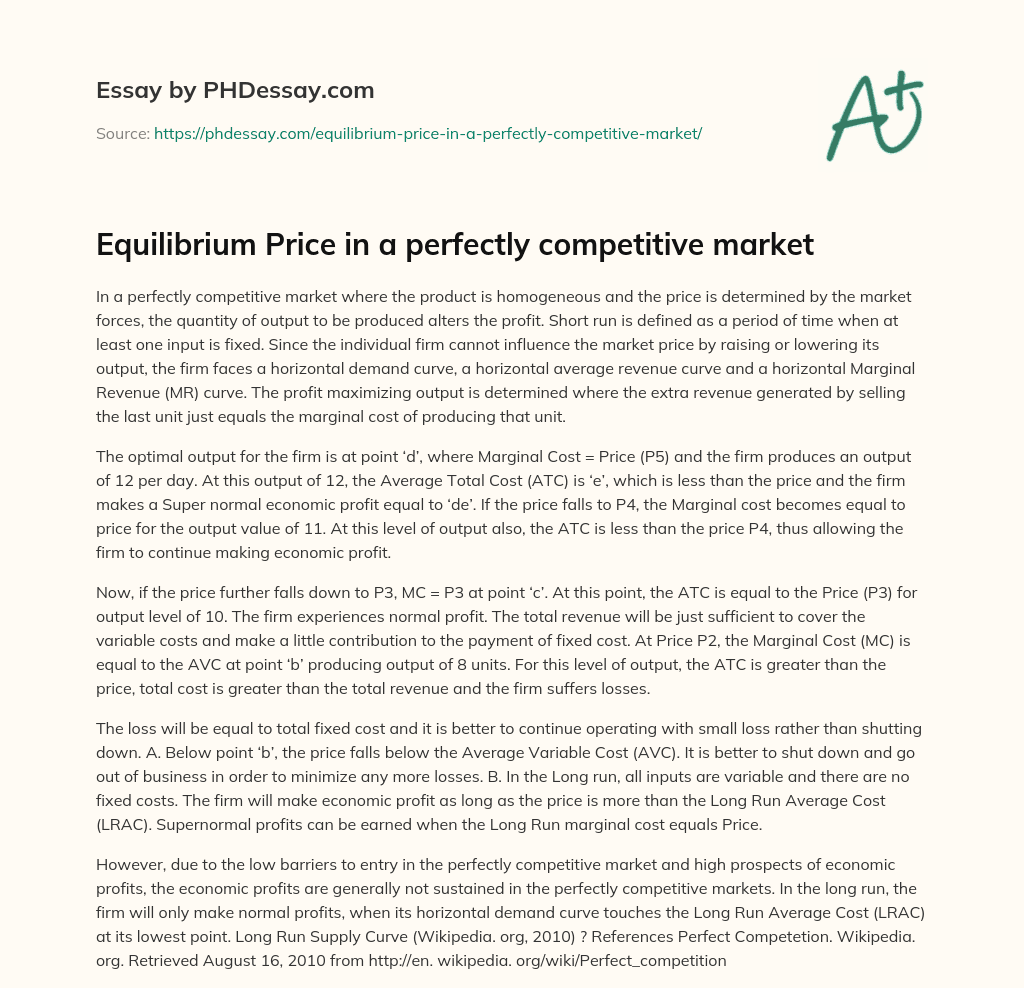Last Updated 27 Jul 2020

# Equilibrium Price in a perfectly competitive market

Words 482 (1 page)
Views 243

In a perfectly competitive market where the product is homogeneous and the price is determined by the market forces, the quantity of output to be produced alters the profit. Short run is defined as a period of time when at least one input is fixed. Since the individual firm cannot influence the market price by raising or lowering its output, the firm faces a horizontal demand curve, a horizontal average revenue curve and a horizontal Marginal Revenue (MR) curve. The profit maximizing output is determined where the extra revenue generated by selling the last unit just equals the marginal cost of producing that unit.

The optimal output for the firm is at point ‘d’, where Marginal Cost = Price (P5) and the firm produces an output of 12 per day. At this output of 12, the Average Total Cost (ATC) is ‘e’, which is less than the price and the firm makes a Super normal economic profit equal to ‘de’. If the price falls to P4, the Marginal cost becomes equal to price for the output value of 11. At this level of output also, the ATC is less than the price P4, thus allowing the firm to continue making economic profit.

Order custom essay Equilibrium Price in a perfectly competitive market with free plagiarism report

GET ORIGINAL PAPER

Now, if the price further falls down to P3, MC = P3 at point ‘c’. At this point, the ATC is equal to the Price (P3) for output level of 10. The firm experiences normal profit. The total revenue will be just sufficient to cover the variable costs and make a little contribution to the payment of fixed cost. At Price P2, the Marginal Cost (MC) is equal to the AVC at point ‘b’ producing output of 8 units. For this level of output, the ATC is greater than the price, total cost is greater than the total revenue and the firm suffers losses.

The loss will be equal to total fixed cost and it is better to continue operating with small loss rather than shutting down. A. Below point ‘b’, the price falls below the Average Variable Cost (AVC). It is better to shut down and go out of business in order to minimize any more losses. B. In the Long run, all inputs are variable and there are no fixed costs. The firm will make economic profit as long as the price is more than the Long Run Average Cost (LRAC). Supernormal profits can be earned when the Long Run marginal cost equals Price.

However, due to the low barriers to entry in the perfectly competitive market and high prospects of economic profits, the economic profits are generally not sustained in the perfectly competitive markets. In the long run, the firm will only make normal profits, when its horizontal demand curve touches the Long Run Average Cost (LRAC) at its lowest point. Long Run Supply Curve (Wikipedia. org, 2010) ? References Perfect Competetion. Wikipedia. org. Retrieved August 16, 2010 from http://en. wikipedia. org/wiki/Perfect_competitionThis essay was written by a fellow student. You can use it as an example when writing your own essay or use it as a source, but you need cite it.

## Get professional help and free up your time for more important courses

Starting from 3 hours delivery 450+ experts on 30 subjects
get essay help 124  experts online

Did you know that we have over 70,000 essays on 3,000 topics in our database?